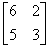### Definition of Non-singular Matrix

If the determinant of a matrix is not equal to zero, then the matrix is called a non-singular matrix.

An n x n(square) matrix A is called non-singular if there exists an n x n matrix B such that AB = BA = In, where In, denotes the n x n identity matrix.

If the matrix is non-singular, then its inverse exists.

Properties of non-singular matrix:

1. If A and B are non-singular matrices of the same order, then AB is non-singular.
2. If A is non-singular, then Ak is non-singular for any positive integer k.
3. If A is non-singular and k is a non-zero scalar, then kA is non-singular.

### Example of Non-singular Matrix

The determinant ofi.e.= 6(3) – 5(2) = 18 - 10 = 8 ≠ 0, so it is a non-singular matrix.

• N/A